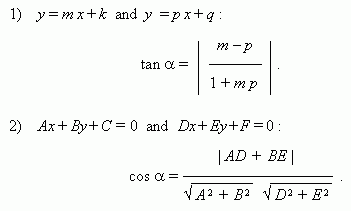Straight line

General equation of straight line. Normal vector.

Equation of straight line with slope.

Equation of straight line in segments on axes.

Equation of straight line going through two different points.

Parametric equation of straight line.

Parallelism condition of straight lines.

Perpendicularity condition of straight lines.

Distance between two points .

Distance from point to straight line.

Distance between parallel straight lines.

Angle between straight lines.

A general equation of straight line:

Àõ +  Âó +  Ñ = 0 ,

where À and Â aren't equal to zero simultaneously.

Coefficients À and Â are coordinates of normal vector of the straight line ( i.e. vector, perpendicular to the straight line ). At À = 0  straight line is parallel to the axis ÎÕ , at Â = 0 straight line is parallel to the axis Î Y .

At Â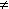0 we receive an equation of straight line with a slope :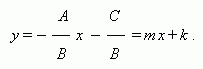An equation of the straight line, going through the point ( õ 0 , ó 0 ) and not parallel to the axis OY :

ó ó 0 = m ( x õ 0 ) ,

where m is a slope , equal to tangent of an angle between the straight line and the positive direction of the axis ÎÕ .

At À0, Â0 and Ñ0 we receive an equation of straight line in segments on axes :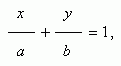where a = – C / A , b = – C / B . This line goes through the points ( a , 0 ) and ( 0, b ), i.e. it cuts off segments a and b long on the coordinate axes.

An equation of straight line going through two different points ( õ 1 , ó 1 ) and ( õ 2 , ó 2 ):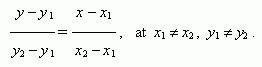A parametric equation of straight line , going through a point ( õ 0 , ó 0 ) and parallel to a direction vector ( a , b ) of a straight line :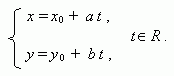A parallelism condition of straight lines:

1)  for lines Àõ + Âó + Ñ = 0  and D õ + E ó + F = 0 : AE BD = 0 ,

2)  for lines ó = m x+ k and ó = p x+ q : m = p .

A perpendicularity condition of straight lines:

1)  for lines Àõ + Âó + Ñ = 0  and D õ + E ó + F = 0 : AD + BE = 0 ,

2)  for lines ó = m x+ k and ó = p x+ q : m p = – 1 .

A distance between two points ( x 1 , y 1 ) and ( x 2 , y 2 ) :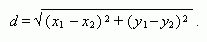A distance from a point ( õ 0 , ó 0 ) to a straight line Àõ + Âó + Ñ = 0 :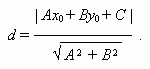A distance between parallel straight lines Àõ + Âó + Ñ = 0 and A õ + B ó + F = 0 :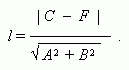An angle a between straight lines: